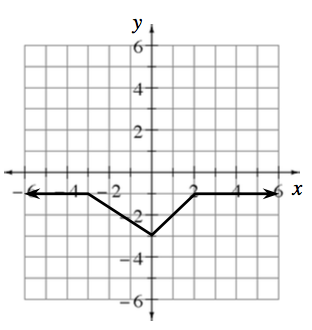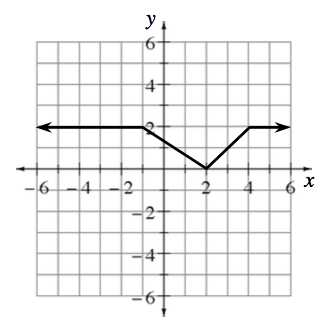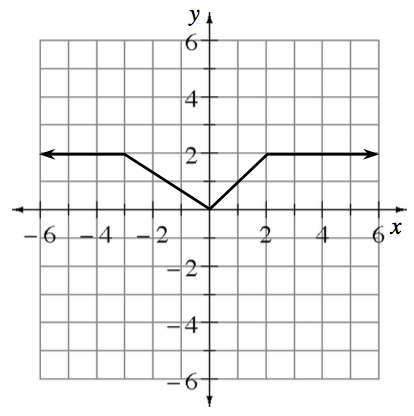### Home > INT2 > Chapter 12 > Lesson 12.1.3 > Problem12-41

12-41.

Given the graph of $y = f(x)$ at right, write an equation in the form $y = f(x - h) + k$ for each transformation.

When dealing with translations, remember that the number outside the parentheses affects the graph vertically, and the number inside the parentheses affects the graph horizontally.

1.$y = f(x) - 3$

1.